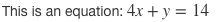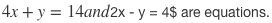## QUIZALIZE ПРЕВРАЩАЕТ ТЕСТЫ В ИГРУ

Quizalize — хороший способ превратить проверочную работу в увлекательную игру. Начать работу с бесплатным онлайн-сервисом можно после регистрации: сочинять вопросы для тестов или выбирать их… Read More »QUIZALIZE ПРЕВРАЩАЕТ ТЕСТЫ В ИГРУ## Math Mode on Quizalize

Writing equations, fractions or other complex formulas on a computer can be a terribly dull affair. There are a few words with which to describe the equation editor in Microsoft Word, but none are appropriate for audiences of all ages!

Thankfully, Quizalize features a super simple and quick way to include good-looking formulas in your questions and answers. Here’s an example:All you need to do is to click the Math Mode button, in the upper right corner. The question input screen will then show live preview boxes. As you enter your formulas in the super-straightforward LaTex format (more on this below), these boxes automatically update so you can easily check your work.Quizalize is built to handle LaTex format equations. It’s actually rather simple. All you need to do is include your formula in between two \$ symbols. Here’s a quick example.

Writing this:

This is an equation: \$4x + y = 14\$

Turns into this:As you can see, the text in between the dollar symbols was transformed into a nice-looking equation. The rest of the text was not. It is important to remember to include the second \$, otherwise it will not work.

It is possible to include 2 or more separate equations with text in-between. All you need to do is remember to enclose each part with \$ symbols.

Writing this:

\$4x + y = 14\$ and \$2x – y = 4\$ are equations.

Turns into this:Because the “and” was not included inside the \$ symbols, it is kept as text. However, let’s see what happens when we forget a \$.

Writing this:

\$4x + y = 14 and \$2x – y = 4\$ are equations.

Turns into this:As you can see, the “and” is now part of the first equation. The second equation is no longer transformed as its initial \$ is now closing the first one. But, not to worry! Because the live preview boxes update as you write, it’s really easy to notice and fix.

Read More »Math Mode on Quizalize